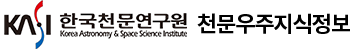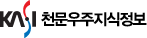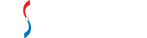통합검색

통합검색

인기검색어논문 상세보기

• 홈으로 이동
ESCAPE OF RESONANTLY SCATTERED LYβ AND Hα FROM HOT AND OPTICALLY THICK MEDIA
• 저자
Chang, Seok-Jun
• 저널명
The Korean Astronomical Society
• 발행년도
2018
• 권호
V .51, N .1
• 원문보기
We investigate the escape of $Ly{\beta}$ from emission nebulae with a significant population of excited hydrogen atoms in the level n = 2, rendering them optically thick in $H{\alpha}$ . The transfer of $Ly{\beta}$ line photons in these optically thick regions is complicated by the presence of another scattering channel leading to re-emission of $H{\alpha}$ , alternating their identities between $Ly{\beta}$ and $H{\alpha}$ . In this work, we develop a Monte Carlo code to simulate the transfer of $Ly{\beta}$ line photons incorporating the scattering channel into $H{\alpha}$ . Both $H{\alpha}$ and $Ly{\beta}$ lines are formed through diffusion in frequency space, where a line photon enters the wing regime after a fairly large number of resonance scatterings with hydrogen atoms. Various line profiles of $H{\alpha}$ and $Ly{\beta}$ emergent from our model nebulae are presented. It is argued that the electron temperature is a critical parameter which controls the flux ratio of emergent $Ly{\beta}$ and $H{\alpha}$ . Specifically for $T\;=\;3{\times}10^4\;K$ and $H{\alpha}$ line center optical depth $\tau{\alpha}\;=\;10$ , the number flux ratio of emergent $Ly{\beta}$ and $H{\alpha}$ is ~ 49 percent, which is quite significant. We propose that the leaking $Ly{\beta}$ can be an interesting source for the formation of $H{\alpha}$ wings observed in many symbiotic stars and active galactic nuclei. Similar broad $H{\alpha}$ wings are also expected in $Ly{\alpha}$ emitting halos found in the early universe, which can be potentially probed by the James Webb Telescope in the future.